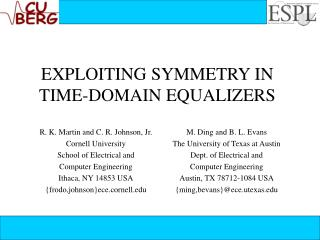Download PresentationEXPLOITING SYMMETRY IN TIME-DOMAIN EQUALIZERSEXPLOITING SYMMETRY IN TIME-DOMAIN EQUALIZERS - PowerPoint PPT Presentation

Download PresentationEXPLOITING SYMMETRY IN TIME-DOMAIN EQUALIZERS
An Image/Link below is provided (as is) to download presentation

Download Policy: Content on the Website is provided to you AS IS for your information and personal use and may not be sold / licensed / shared on other websites without getting consent from its author. While downloading, if for some reason you are not able to download a presentation, the publisher may have deleted the file from their server.

- - - - - - - - - - - - - - - - - - - - - - - - - - - E N D - - - - - - - - - - - - - - - - - - - - - - - - - - -
Presentation Transcript

1. EXPLOITING SYMMETRY IN TIME-DOMAIN EQUALIZERS M. Ding and B. L. Evans The University of Texas at Austin Dept. of Electrical and Computer Engineering Austin, TX 78712-1084 USA {ming,bevans}@ece.utexas.edu R. K. Martin and C. R. Johnson, Jr. Cornell University School of Electrical and Computer Engineering Ithaca, NY 14853 USA {frodo,johnson}ece.cornell.edu

2. channel carrier magnitude subchannel frequency Subchannels are 4.3 kHz wide in ADSL Introduction • Discrete Multitone (DMT) Modulation • Multicarrier: Divide Channel into Narrow band subchannels • Band partition is based on fast Fourier transform (FFT) • Standardized for Asymmetric Digital Subscriber Lines (ADSL)

3. N/2 subchannels N real samples S/P quadrature amplitude modulation (QAM) encoder mirror data and N-IFFT add cyclic prefix P/S D/A + transmit filter Bits TRANSMITTER channel RECEIVER N/2 subchannels N real samples P/S time domain equalizer (TEQ) QAM demoddecoder N-FFT and remove mirrored data S/P remove cyclic prefix receive filter + A/D Up to N/2 1 - tap FEQs ADSL Transceiver (ITU Structure)

4. copy copy s y m b o l ( i+1) CP CP s y m b o li CP: Cyclic Prefix v samples N samples channel Shortened channel Cyclic Prefix Combats ISI with TEQ • CP provides guard time between successive symbols • We use finite impulse response • (FIR) filter called a time domain • equalizer to shorten the channel • impulse response to be no longer than cyclic prefix length

5. nk yk rk xk h w + MSSNR TEQ Design • Maximum Shortening SNR (MSSNR) TEQ: Choose w to minimize energy outside window of desired length • The design problem is stated as • The solution will be the generalized eigenvector corresponding to the largest eigenvalue of matrix pencil (B, A) hwin, hwall : equalized channel within and outside the window

6. Symmetry in MSSNR designs • Fact: eigenvectors of a doubly-symmetric matrix are symmetric or skew-symmetric. • MSSNR solution: • A and B are almost doubly symmetric • For long TEQ lengths, w becomes almost perfectly symmetric • MSSNR for Unit Norm TEQ (MSSNR-UNT) solution: • A is almost doubly symmetric • In the limit, the eigenvector of A converge to the eigenvector of HTH, which has symmetric or skew-symmetric eigenvectors.

7. Symmetric MSSNR TEQ design • Idea: force the TEQ to be symmetric, and only compute half of the coefficients. • Implementation: instead of finding an eigenvector of an Lteq  Lteqmatrix, we only need to find an eigenvector of an matrix. • The phase of a perfectly symmetric TEQ is linear, • Achievable bit rates:

8. Matlab DMTTEQ Toolbox 3.1 • The symmetric design has been implemented in DMTTEQ toolbox. • Toolbox is a test platform • for TEQ design and • performance evaluation. • Most popular algorithms • are included in the toolbox • Graphical User Interface: • easy to customize your • own design. • Available at http://www.ece.utexas.edu/~bevans/projects/adsl/dmtteq/

9. Conclusions • Infinite length MMSNR TEQs with a unit norm constraint are exactly symmetric, while finite length MSSNR TEQs are approximately symmetric. • A symmetric MSSNR TEQ only has one fourth of FIR implementation complexity. • Symmetric design enables frequency domain equalizer and TEQ to be trained in parallel. • Symmetric design exhibits only a small loss in the bit rate over non-symmetric MSSNR TEQs. • Symmetric design doubles the length of the TEQ that can be designed in fixed point arithmetic.